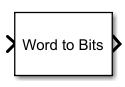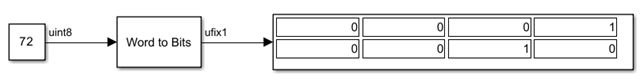# Word to Bits

Converts real numbers to vector of bits

Since R2023a

•Libraries:
HDL Coder / Logic and Bit Operations

## Description

The Word to Bits block converts a real number of word length N to a vector of bits. The output of the block is a vector whose length you specify in the Maximum Word length parameter.

The block treats the first element of the output vector as the least significant bit (LSB) and treats subsequent bits as the next significant bits in ascending order.

This figure shows the conversion of the unsigned integer 72 to its binary representation when you specify a maximum word length of 8. You can also convert a signed integer value to its corresponding binary vector.This figure shows the conversion of the non-integer value 5.5 to its binary representation. The output is a binary representation of the stored integer value of 5.5. To determine stored integer value of a fixed-point real number, use `storedInteger` function.## Limitations

The block has these limitations:

• Floating-point input is not supported.

• Bus, vector, and matrix inputs are not supported.

• Complex input is not supported.

## Ports

### Input

expand all

Input signal, specified as a scalar. The word-length must be less than or equal to the Maximum Word Length parameter.

Example: `72`

Example: `5.5`

Data Types: `int8` | `int16` | `int32` | `int64` | `uint8` | `uint16` | `uint32` | `uint64` | `fixed point`

### Output

expand all

Output signal, returned as a binary-valued scalar or vector. Set the length of this vector in theMaximum Word length parameter. The block treats the first element of the output vector as the LSB and treats subsequent bits as the next significant bits in ascending order.

Data Types: `ufix1`

## Parameters

expand all

Maximum output word length, specified as an integer in the range [1, 128]. The parameter value must be greater than or equal to the input signal word-length.

#### Programmatic Use

 Block parameter: `maxWordLength` Type: character vector Value: Integer in the range [1, 128] Default: `'8'`

## Version History

Introduced in R2023a